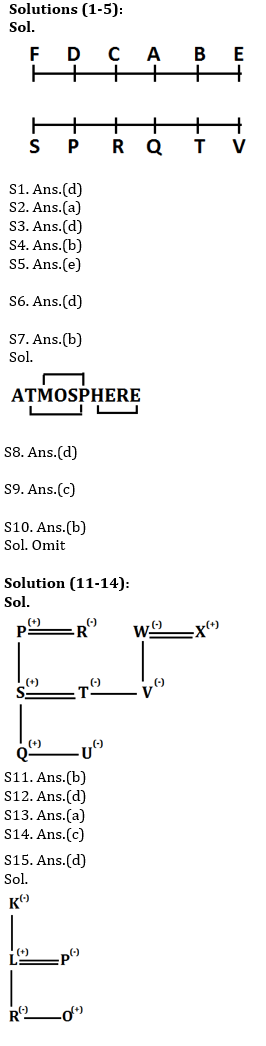Latest Banking jobs   »   Reasoning Ability, Blood Relation Quiz For...

# Reasoning Ability, Quiz For SBI PO, Clerk Prelims 2021- 19th June

Directions (1-5): Study the following information carefully and answer the questions given below:
Twelve people are sitting in two parallel rows containing six people each, in such a way that there is an equal distance between adjacent persons. In row–1 A, B, C, D, E and F are seated and all of them are facing south. In row-2 P, Q, R, S, T and V are seated and all of them are facing north. Therefore, in the given seating arrangement each member seated in a row faces another member of the other row.
P sits third to the left of T. Neither P nor T sits at an extreme end of the line. A sits second to the right of E. Neither A nor E faces T or P. A does not sit at an extreme end. R does not face A and R does not sit at an extreme end of the line. Only one person sits between F and C. Neither F nor C faces T. C does not sit at the extreme end. Only one person sits between V and Q. F is not an immediate neighbour of B. A does not face V.

Q1. How many people sit to the right of V?
(a) One
(b) Two
(c) Three
(d) No one
(e) Five

Q2. V is related to B in the same way as Q is related to C. Which of the following is related to P, following the same pattern?
(a) F
(b) A
(c) D
(d) E
(e) None of these

Q3. Which of the following is true regarding V?
(a) V faces A
(b) T is not an immediate neighbour of V.
(c) C faces the one who is second to left of V
(d) V sits at one of the extreme ends of the line
(e) R sits third to the right of V

Q4. Who among the following sit at extreme ends of the rows?
(a) D, F
(b) V, S
(c) Q, S
(d) B, D
(e) None of these

Q5. Who among the following faces B?
(a) V
(b) S
(c) Q
(d) R
(e) T

Q6. Four of the following are alike in a certain way so form a group, which among the following does not belong to that group?
(a) CEHL
(b) PRUY
(c) FHKO
(d) QSUX
(e) JLOS

Q7. How many pairs of letters are there in the word ‘ATMOSPHERE’, each of which as many letters between then in the word have as they have between then in the English alphabet?
(a) One
(b) Three
(c) Two
(d) Four
(e) None

Q8. If in the word GEOGRAPHY, all vowels are replaced by its next letter and all consonant is replaced by its previous letter, then all letters are arranged in alphabetical order from left to right. Then which of the following letter fourth from the right end?
(a) P
(b) B
(c) G
(d) O
(e) F

Q9. If the number ‘53982764’, One is subtracted in odd digit and one is added in even digit of the number then numbers are arranged in ascending order from left to right. Then what is the sum of the digit which is third from the left end and the digit which is fourth from the right end?
(a) 8
(b) 12
(c) 10
(d) 5
(e) None of these

Q10. How many words can be formed from the 1st, 3rd, 6th and 8th letter of a word ‘OPTIMISM’ by using each letter once in the word?
(a) Three
(b) One
(c) None
(d) Two
(e) More than three

Directions (11-14): Study the following information and answer the given questions.
There are nine members in three generations and three married couples in the family. There are only four male members in the family. Q is son of T who is daughter-in-law of P. X is father of V. R is mother of S who is father of U. S is son-in-law of W. V is unmarried and aunt of U. X is not married to R.

Q11. Who among the following is Son-in law of X?
(a) P
(b) S
(c) Q
(d) R
(e) U

Q12. How R is related to Q?
(a) Grandfather
(b) Father
(c) Sister
(d) Grandmother
(e) Brother

Q13. Who among the following is nephew of V?
(a) Q
(b) S
(c) R
(d) P
(e) T

Q14. Which of the statement is false with respect to T?
(a) T is mother of U
(b) P is father-in-law of T
(c) X is mother of T
(d) T is sister of V
(e) All are true

Q15. If there are 5 members in a family, K is mother-in-law of P. L is father of R. O is son of P. R is unmarried female. How K related to O?
(a) Mother
(b) Father
(c) Aunt
(d) Grandmother
(e) Sister

Practice More Questions of Reasoning for Competitive Exams:

###### SBI Clerk Study Plan 2021

Solutions